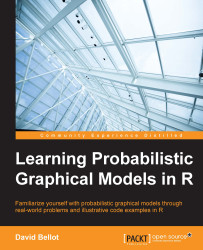•#### Learning Probabilistic Graphical Models in R#### Overview of this book

Probabilistic graphical models (PGM, also known as graphical models) are a marriage between probability theory and graph theory. Generally, PGMs use a graph-based representation. Two branches of graphical representations of distributions are commonly used, namely Bayesian networks and Markov networks. R has many packages to implement graphical models. We’ll start by showing you how to transform a classical statistical model into a modern PGM and then look at how to do exact inference in graphical models. Proceeding, we’ll introduce you to many modern R packages that will help you to perform inference on the models. We will then run a Bayesian linear regression and you’ll see the advantage of going probabilistic when you want to do prediction. Next, you’ll master using R packages and implementing its techniques. Finally, you’ll be presented with machine learning applications that have a direct impact in many fields. Here, we’ll cover clustering and the discovery of hidden information in big data, as well as two important methods, PCA and ICA, to reduce the size of big problems.
Learning Probabilistic Graphical Models in RCreditswww.PacktPub.comPrefaceFree Chapter
Probabilistic ReasoningExact InferenceLearning ParametersBayesian Modeling – Basic ModelsApproximate InferenceBayesian Modeling – Linear ModelsProbabilistic Mixture ModelsAppendixIndex## Summary

In this last chapter, we saw more advanced probabilistic graphical models, whose solution is not easy to compute with standard tools such as the junction tree algorithm. This chapter set out to show that the graphical model framework can still be used even if one has to develop a special algorithm for each model. Indeed, in the LDA model, the solution to the variational problem appeared by looking at the graph of the original LDA and by transforming this graph, thus leading to a better approximation of the initial problem. So, even if the final algorithm does not use the graph directly like a junction tree algorithm would do, the solution came from the graph anyway.

This chapter proved how powerful probabilistic graphical models can be, and all the possibilities and new models that can be created from simpler models.

Indeed, each of these models can again be extended either by combining them—for example, in the mixture of experts model. In this model, each expert function could be...